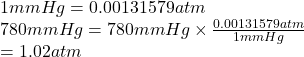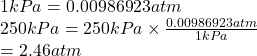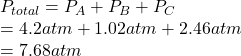## Find the total pressure (in atm) of a mixture that contains 3 gases with the following partial pressures. Gas A is 4.2atm, Gas B is 780 mmHg

Question

Find the total pressure (in atm) of a mixture that contains 3 gases with the following partial pressures. Gas A is 4.2atm, Gas B is 780 mmHg, Gas C is 250.00kPa

in progress 0
3 months 2021-08-15T07:51:18+00:00 1 Answers 0 views 0

1. Answer: The total pressure of given mixture that contains 3 gases is 7.68 atm.

Explanation:

Given: Partial pressure of gases is:

Gas A = 4.2 atm

Gas B = 780 mm Hg

Convert mm Hg into atm is as follows.Gas C = 250 kPa

Convert kPa into atm as follows.Total pressure is the sum of partial pressures of gases present in the mixture.

Therefore, total pressure of given mixture is calculated as follows.Thus, we can conclude that the total pressure of given mixture that contains 3 gases is 7.68 atm.